# AP Board 6th Class Maths Solutions Chapter 4 Integers Ex 4.2

AP State Syllabus AP Board 6th Class Maths Solutions Chapter 4 Integers Ex 4.2 Textbook Questions and Answers.

## AP State Syllabus 6th Class Maths Solutions 4th Lesson Integers Ex 4.2

Question 1.
Put appropriate symbol > or < in the boxes given.
i) -1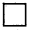0
ii) -3-7
iii) -10+10
i) -1 < 0
ii) -3 > -7
iii) -10 < +10Question 2.
Write the following integers in increasing and decreasing order:
i) -7, 5, -3
ii) -1, 3, 0
iii) 1, 3, -6
iv) -5, – 3, -1

 S.No. Numbers Increasing order Decreasing order i) -7, 5, -3 -7 < 5 < -3 5 > -3 > -7 ii) -1, 3, 0 -1 < 0 < 3 3 > 0 > -1 iii) 1, 3, -6 -6 < 1 < 3 3 > 1 > -6 iv) -5, -3, -1 -5 < -3 < -1 -1 > -3 > -5

Question 3.
Write True or False.
i) Zero is on the right of -3 ( )
ii) -12 and +12 represent on the number line the same integer ( )
iii) Every positive integer is greater than zero ( )
iv) (-100) > (+100) ( )
i) True
ii) False
iii) True
iv) FalseQuestion 4.
Find all integers which lie between the given two integers. Represent them on number line:
i) -1 and 1
ii) -5 and 0
iii) -6 and -8
iv) 0 and -3
i) Given integers -1 and +1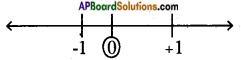Integers between -1 and 1 is 0.

ii) Given integers -5 and 0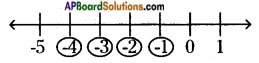Integers between -5 and 0 are -4, -3, -2, -1.

iii) Given, integers -6 and -8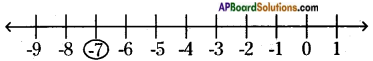Integers between -6 and -8 is -7.

iv) Given integers 0 and -3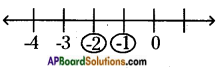Integers between 0 and -3 are -1, -2.Question 5.
The temperature recorded in Shimla is -4°C and in Kufri is -6°C on the same day. Which place is colder on that day? Why?